Courses

# Push Down Automata: CFL & DCFL (Basic Level) - 1

## 10 Questions MCQ Test Question Bank for GATE Computer Science Engineering | Push Down Automata: CFL & DCFL (Basic Level) - 1

Description
This mock test of Push Down Automata: CFL & DCFL (Basic Level) - 1 for Computer Science Engineering (CSE) helps you for every Computer Science Engineering (CSE) entrance exam. This contains 10 Multiple Choice Questions for Computer Science Engineering (CSE) Push Down Automata: CFL & DCFL (Basic Level) - 1 (mcq) to study with solutions a complete question bank. The solved questions answers in this Push Down Automata: CFL & DCFL (Basic Level) - 1 quiz give you a good mix of easy questions and tough questions. Computer Science Engineering (CSE) students definitely take this Push Down Automata: CFL & DCFL (Basic Level) - 1 exercise for a better result in the exam. You can find other Push Down Automata: CFL & DCFL (Basic Level) - 1 extra questions, long questions & short questions for Computer Science Engineering (CSE) on EduRev as well by searching above.
QUESTION: 1

### Which of the following is not true?

Solution: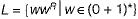cannot be accepted by the deterministic PDA since guessing of the alphabet after which reversal is done is required. One must have to guess the end of w, so that start of wR is found. The word cannot be determined deterministically as w ∈ (0 + 1)* which includes multiple combinations.

QUESTION: 2

### Which of the following statements are true? (i) The complement of a language is always regular. (ii) The intersection of regular languages is regular. (iii) The complement of a regular language is regular.

Solution:

According to the closure properties regular language is closed under union, intersection, Kleen closure, concatenation, complementation. But the complementation of any language need not to be always regular.

QUESTION: 3

### Which of the following is not true?

Solution:

According to closure properties regular languages are closed under the ail finite basic operations like: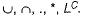But CFL is closed only under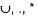Also Regular inter section of CFL i.e. CFL intersected with regular language.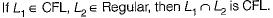QUESTION: 4

Context-free languages and regular languages are both closed under the operations(s) of
(i) Union
(ii) Intersection
(iii) Concatenation

Solution:

Regular and context free languages are both closed under union and concatenation while only regular is closed under intersection.

QUESTION: 5

Which of the following languages are context free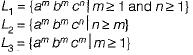Solution:

In L1 only one stack is required since only one comparison is there. While for Land Ltwo stacks are required since there are 2 comparisons. So, only L1 is context free language as it is accepted by PDA.

QUESTION: 6

If L1, is regular and L2 is CFL over ∑* which of the following statement is incorrect?

Solution:

Since L1 and L2 are regular and context free languages respectively CFL are closed under regular ∪, ∩, therefore
(a) L1 ∪ L2 is CFL and therefore regular too.
(b) L1 ∩ L2 is not regular
(c) L1 * is regular

QUESTION: 7

Context free languages are closed under

Solution:

Out of the four operations given, as per the closure properties CFL are closed under only union.

QUESTION: 8

Which of the following languages is/are context free?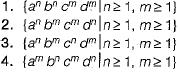Solution:

Here for the languages (i) and (ii) we can design a PDA Hence they are CFL.
In (iii) and (iv) we cannot conclude whether number of (a) = number of (c) and number of (b) = number of (d) using only one stack memory so they aren’t CFL.

QUESTION: 9

Which of the following is undecidable?

Solution:
QUESTION: 10

Context-free language can be recognized by

Solution:

Since the power of recognization of NFA is less then the PDA. Also CFL can be recognized by the one which has equal or higher power than the PDA. Both PDA and LBA can recognize the CFL.